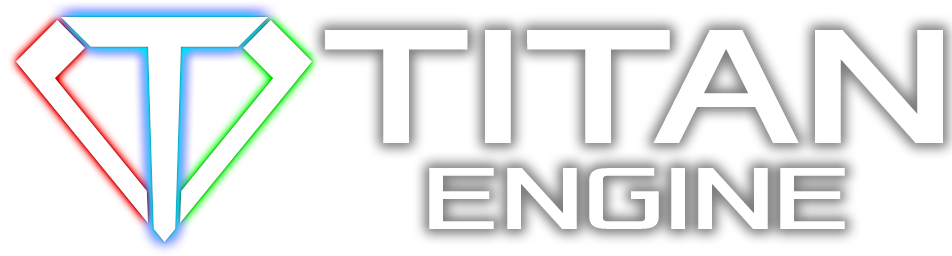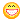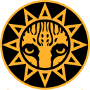About Store Forum Documentation ContactSliding GUI object Author Message
KiekosMember

 Post: #1 Sliding GUI object Hey guys! I've got another problem... There's way too many of 'em... :/ I wanted to have one of the GUI objects slide from the side of the screen when I want it visible and then hide by sliding out of the screen again. The problem is that I've tried a couple of methods and they either do absolutely nothing or crash the whole gameThe one I've tried first (that does absolutely nothing): Code: ```Flt desired_pos_x = -D.w() - questbar.getWindow("questbar").size().x; Lerp(questbar.getWindow("questbar").pos().x, desired_pos_x, 0.1f);``` The second and other ones similiar to this one (which crash the game): Code: ```Flt current_pos_x =          questbar.getWindow("questbar").pos() .x; Flt desired_pos_x = -D.w() - questbar.getWindow("questbar").size().x; while(current_pos_x != desired_pos_x) {     current_pos_x -= 0.05f;     questbar.getWindow("questbar").pos(Vec2(current_pos_x,questbar.getWindow("questbar").pos().y)); }``` Any help will be much appreciated! Thanks, Kiekos
11-15-2013 01:16 PM
PheraelMemberPost: #2 RE: Sliding GUI object Lerp doesn't work this way. Check description. Also, in second code, your loop will be infinite, use something like this instead: Code: ```Flt current_pos_x =          questbar.getWindow("questbar").pos() .x; Flt desired_pos_x = -D.w() - questbar.getWindow("questbar").size().x; while(current_pos_x > desired_pos_x) {     current_pos_x -= 0.05f;     questbar.getWindow("questbar").pos(Vec2(current_pos_x,questbar.getWindow("questbar").pos().y)); }``` (This post was last modified: 11-15-2013 08:06 PM by Pherael.)
11-15-2013 08:06 PM
KiekosMember

 Post: #3 RE: Sliding GUI object Well I dunno why wouldn't it work here... There is an instance of Lerp which takes these types of arguments and it works for example when turning my character around (changing angle.x). Allright, the second method doesn't give the efect I wanted. It just hides the window instead of sliding. If not Lerp, then what...? (This post was last modified: 11-15-2013 08:28 PM by Kiekos.)
11-15-2013 08:14 PM
PheraelMemberPost: #4 RE: Sliding GUI object Umm... just slow it. Code: ```Flt current_pos_x =          questbar.getWindow("questbar").pos() .x; Flt desired_pos_x = -D.w() - questbar.getWindow("questbar").size().x; while(current_pos_x > desired_pos_x) {     current_pos_x -= 0.05f * Time.d(); // now sliding speed is const (0.05 per sec), you can change 0.05f to value that give you smooth slide     questbar.getWindow("questbar").pos(Vec2(current_pos_x,questbar.getWindow("questbar").pos().y)); }``` And if you want use Lerp it should look something like this: questbar.getWindow("questbar").pos().x = Lerp(start_pos_x, desired_pos_x, slide_time); where slide_time is cur slide time (not total) for example: //init this somewhere slide_time_total = 1.0f slide time = 0.0f and in your loop if(slide_time
11-15-2013 08:57 PM
KiekosMember

 Post: #5 RE: Sliding GUI object Code: `current_pos_x -= 0.05f * Time.d(); // now sliding speed is const (0.05 per sec), you can change 0.05f to value that give you smooth slide` I'm sorry to say that it doesn't slow the action. It does the same even with very little values. Just *pop* and it's gone, no sliding. EDIT: Look at this: Code: ```if(Kb.b(KB_Q)) {    Vec2   start_pos = questbar.getWindow("questbar").pos();    Vec2 desired_pos = Vec2(-D.w() - questbar.getWindow("questbar").size().x, questbar.getWindow("questbar").pos().y);    questbar.getWindow("questbar").pos( Lerp(start_pos, desired_pos, 0.1f) ); }``` It works if I hold the button. But I'd like to have Kb.bp (pressed) and have the Lerp repeated to finish the motion. And if I put a while() there it stops working at all... Same happens if I use your method with slide_time_total and slide_time. It doesn't do the whole movement, just like 'one bit' of it. (This post was last modified: 11-15-2013 10:32 PM by Kiekos.)
11-15-2013 09:51 PM
PheraelMemberPost: #6 RE: Sliding GUI object Quote:I'm sorry to say that it doesn't slow the action. It does the same even with very little values. Just *pop* and it's gone, no sliding. It's because you do all operations in one frame. Silly me... My bad, I look at your code briefly. Your whole code is wrong. If you want your GUI object slide you need to update it every frame. //do this in some init function Flt current_pos_x = questbar.getWindow("questbar").pos() .x; Flt desired_pos_x = -D.w() - questbar.getWindow("questbar").size().x; // check if button was pressed, then do this in every frame if(current_pos_x > desired_pos_x) { current_pos_x -= 0.05f * Time.d(); // now sliding speed is const (0.05 per sec), you can change 0.05f to value that give you smooth slide questbar.getWindow("questbar").pos(Vec2(current_pos_x,questbar.getWindow("questbar").pos().y)); } (This post was last modified: 11-15-2013 10:51 PM by Pherael.)
11-15-2013 10:49 PM
KiekosMember

 Post: #7 RE: Sliding GUI object Quote:Your whole code is wrong. Oh well... I suck at this, lol. Got it working like I wanted to, thanks!
11-16-2013 03:18 PM
 « Next Oldest | Next Newest »